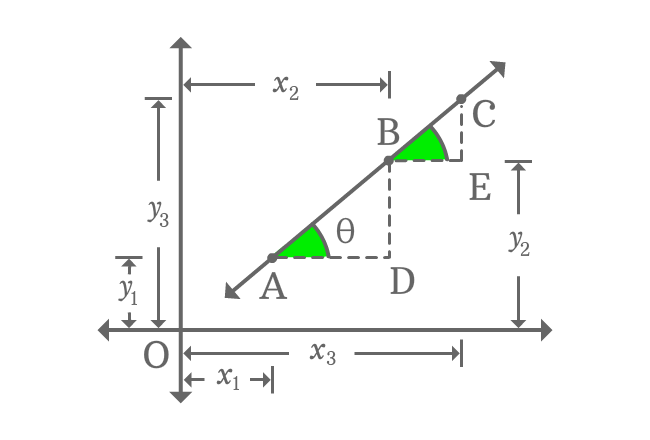# Collinearity of 3 Points

## Formula

$A(x_{1}, y_{1})$, $B(x_{2}, y_{2})$ and $C(x_{3}, y_{3})$ are three points, and the condition for the collinearity of them is

$\dfrac{y_{2}-y_{1}}{x_{2}-x_{1}} \,=\, \dfrac{y_{3}-y_{2}}{x_{3}-x_{2}}$

### Proof

$A(x_{1}, y_{1})$, $B(x_{2}, y_{2})$ and $C(x_{3}, y_{3})$ are three points on a straight line in two dimensional Cartesian coordinate system. The three points are known as collinear points geometrically. Let’s try to derive a condition for the collinearity of three points mathematically.Draw parallel lines to horizontal $x$-axis from points $A$ and $B$. Similarly, draw lines from points $B$ and $C$ but they should be perpendicular to $x$-axis. The parallel and perpendicular lines get intersected at points $D$ and $E$.

It forms two right triangles, known as $\Delta DAB$ and $\Delta EBC$ in two dimensional space.

Geometrically, $\overline{AD} \,\|\, \overline{BE}$ and the two line segments $\overline{AB}$ and $\overline{BC}$ are part of the straight line. Therefore, $\angle DAB$ and $\angle EBC$ are congruent. If $\angle DAB = \theta$, then $\angle EBC = \theta$.

#### According to ΔDAB

Find the slope of the straight line $\overleftrightarrow{AB}$ from right triangle $DAB$.

$\tan{\theta} \,=\, \dfrac{BD}{AD}$

$\implies \tan{\theta} \,=\, \dfrac{OB-OD}{OD-OA}$

$\,\,\, \therefore \,\,\,\,\,\, \tan{\theta} \,=\, \dfrac{y_{2}-y_{1}}{x_{2}-x_{1}}$

#### According to ΔEBC

Find the slope of the straight line $\overleftrightarrow{BC}$ from right triangle $EBC$.

$\tan{\theta} \,=\, \dfrac{EC}{BE}$

$\implies \tan{\theta} \,=\, \dfrac{OC-OE}{OE-OB}$

$\,\,\, \therefore \,\,\,\,\,\, \tan{\theta} \,=\, \dfrac{y_{3}-y_{2}}{x_{3}-x_{2}}$

#### Condition of Collinearity

We know that $\overline{AB}$ and $\overline{BC}$ are part of the straight line $\overleftrightarrow{AC}$. So, the slopes of straight lines $\overleftrightarrow{AB}$ and $\overleftrightarrow{BC}$ are equal.

$\,\,\, \therefore \,\,\,\,\,\, \dfrac{y_{2}-y_{1}}{x_{2}-x_{1}} \,=\, \dfrac{y_{3}-y_{2}}{x_{3}-x_{2}}$

The mathematical equation in algebraic form represents a condition for the collinearity of three points and it is useful to us to verify the collinearity of three or more points mathematically.

Latest Math Topics
Jun 26, 2023
Jun 23, 2023

###### Math Questions

The math problems with solutions to learn how to solve a problem.

Learn solutions

Practice now

###### Math Videos

The math videos tutorials with visual graphics to learn every concept.

Watch now

###### Subscribe us

Get the latest math updates from the Math Doubts by subscribing us.